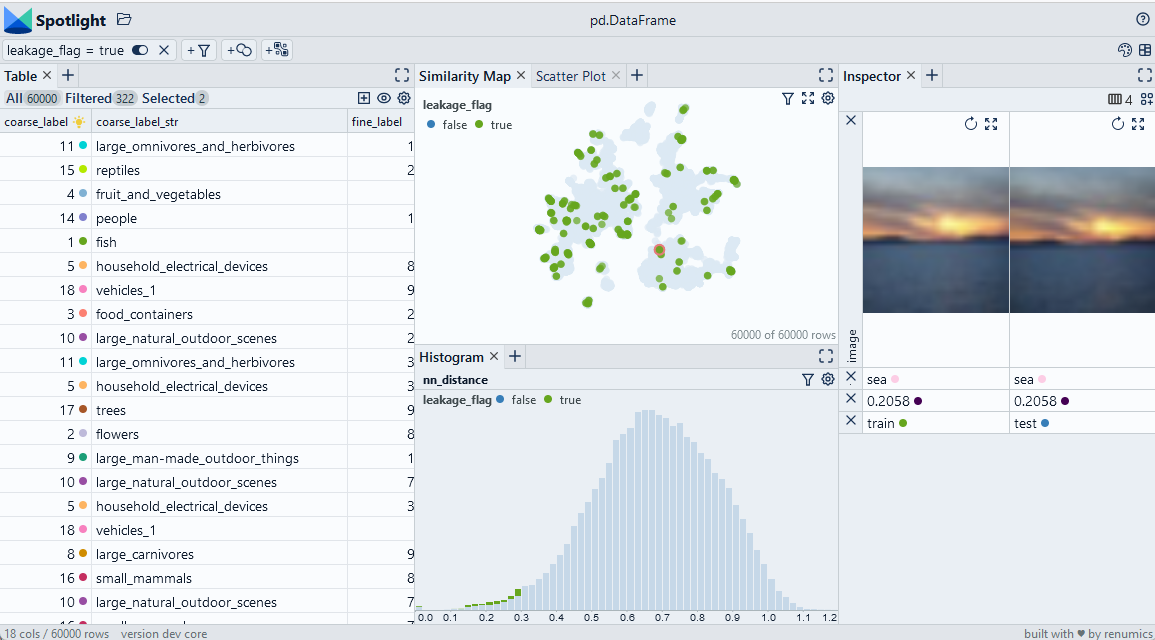🎉 We released a Spotlight OSS Version! ⭐ Star it on Github

Version: 1.0.0

# Detect leakage with Annoy

We use embeddings to detect duplicates by computing nearest neighbors with the Annoy library. Then, we analyse those examples that leak from the training to the validation split. Although the example is based on image embeddings, the basic play is independent of the data type.

Use Chrome to run Spotlight in Colab. Due to Colab restrictions (e.g. no websocket support), the performance is limited. Run the notebook locally for the full Spotlight experience.• `df['nn_id']` contains the sample id for the nearest neighbor in the embedding space.
• `df['split']` contains the name or numeric id for the split for each data sample.
• `df['nn_distance']` contains distance to the nearest neighbor.## Imports and play as copy-n-paste functions​

# Install dependencies
``#@title Install required packages with PIP!pip install renumics-spotlight datasets annoy``
# Play as copy-n-paste functions
``#@title Play as copy-n-paste functionsimport datasetsfrom renumics import spotlightfrom annoy import AnnoyIndeximport pandas as pdimport requestsdef nearest_neighbor_annoy(df, embedding_name='embedding', threshold=0.3, tree_size=100):    embs = df[embedding_name]    t = AnnoyIndex(len(embs), 'angular')    for idx, x in enumerate(embs):          t.add_item(idx, x)    t.build(tree_size)    images = df['image']    df_nn = pd.DataFrame()    nn_id = [t.get_nns_by_item(i,2) for i in range(len(embs))]    df_nn['nn_id'] = nn_id    df_nn['nn_image'] = [images[i] for i in nn_id]    df_nn['nn_distance'] = [t.get_distance(i, nn_id[i]) for i in range(len(embs))]    df_nn['nn_flag'] = (df_nn.nn_distance < threshold)    return df_nndef detect_leakage(df, nn_id_name='nn_id', split_name='split', threshold=0.3):    nn_id = df['nn_id']    split = split = df['split']    df_leak = pd.DataFrame()    df_leak['nn_same_split'] = [True if split[i]==split[nn_id[i]] else False for i in range(len(nn_id))]    df_leak['leakage_flag'] = (df.nn_distance < 0.3) & (df_leak['nn_same_split'] != True )    return df_leak``

## Step-by-step example on CIFAR-100​

### Load CIFAR-100 from Huggingface hub and convert it to Pandas dataframe​

``dataset = datasets.load_dataset("renumics/cifar100-enriched", split="train")df = dataset.to_pandas()``

### Compute heuristics for data leakage based on nearest neighbor distance​

``df_nn = nearest_neighbor_annoy(df)df = pd.concat([df, df_nn], axis=1)df_leak = detect_leakage(df)df = pd.concat([df, df_leak], axis=1)``

### Inspect and remove leakage with Spotlight​

``df_show = df.drop(columns=['embedding', 'probabilities'])layout_url = "https://raw.githubusercontent.com/Renumics/spotlight/playbook_initial_draft/playbook/rookie/leakage_annoy.json"response = requests.get(layout_url)layout = spotlight.layout.nodes.Layout(**json.loads(response.text))spotlight.show(df_show, dtype={"image": spotlight.Image, "embedding_reduced": spotlight.Embedding}, layout=layout)``